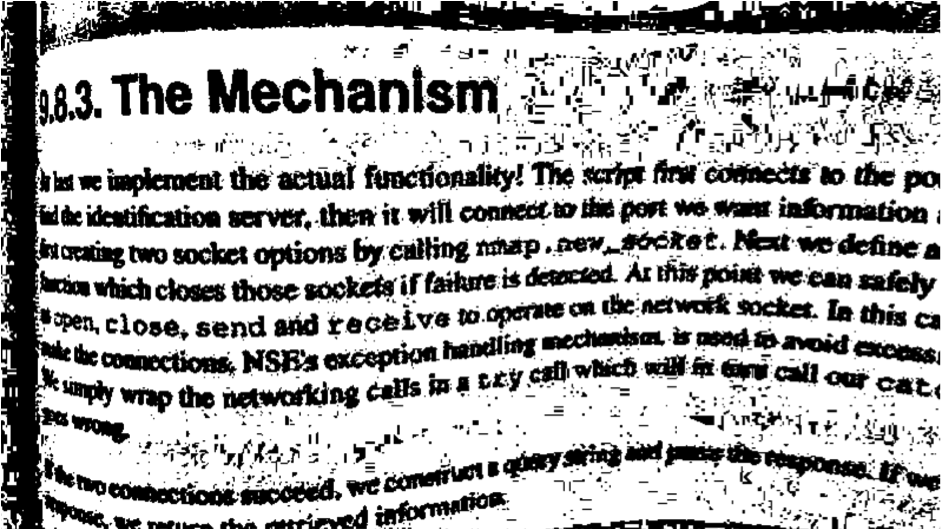# Intro to Image Processing in OpenCV with Python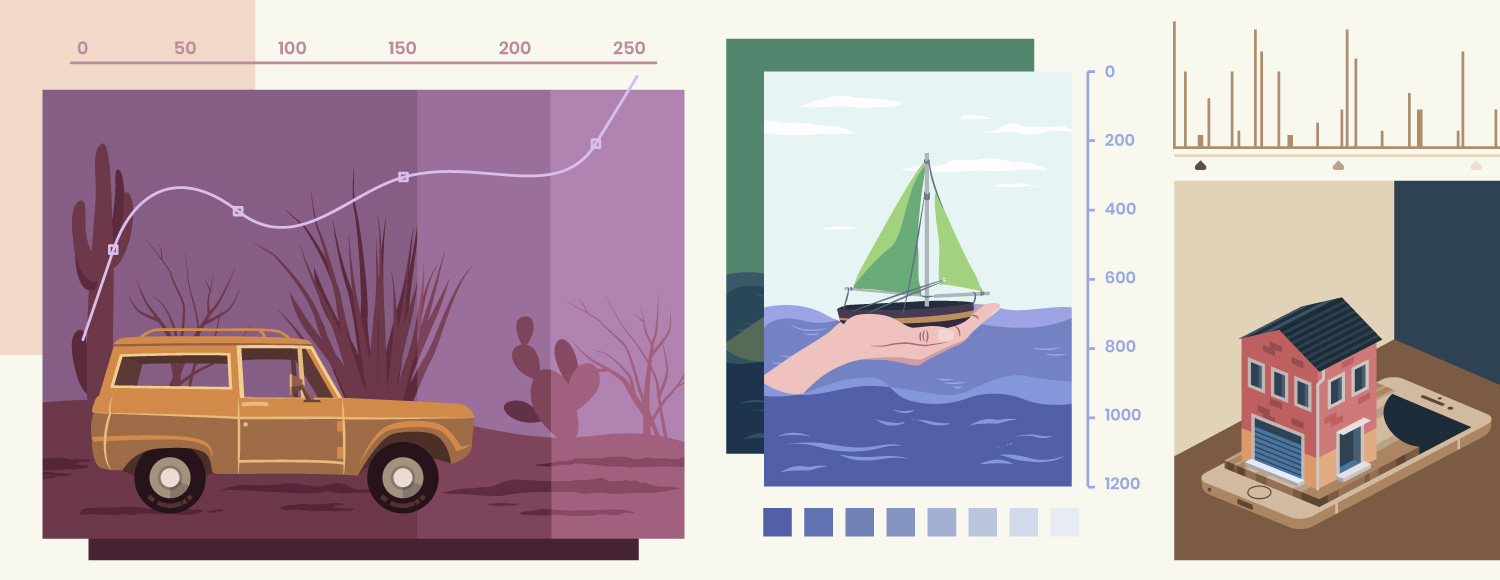Welcome to this tutorial covering OpenCV. This type of program is most commonly used for video and image analysis such as license plate reading, facial recognition, robotics, photo editing and more within C++, Python, C and Java. In this tutorial we’ll cover OpenCV for image analysis.
Firstly, the “CV” part in OpenCV as mentioned in the title stands for “Computer Vision” and Image Processing is just a small part of Computer Vision. Let’s take a few steps back. What is Computer Vision?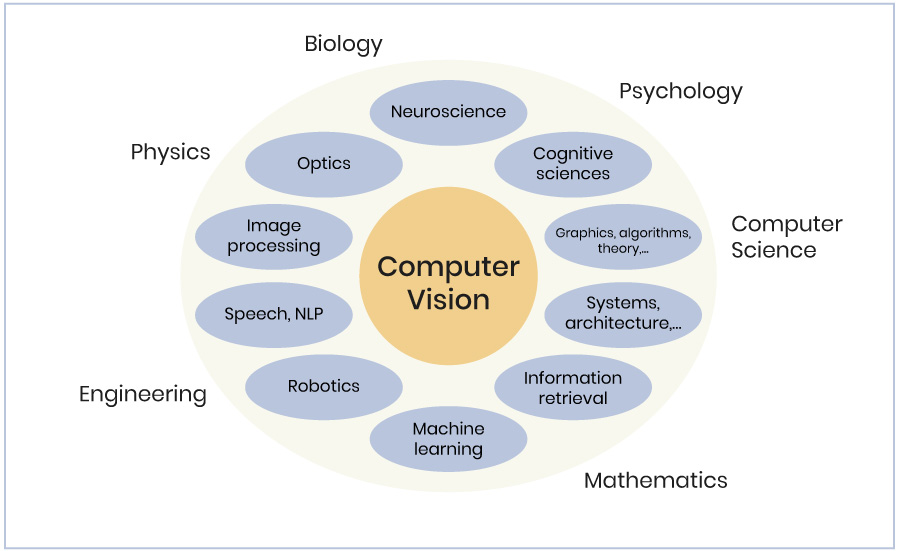As the definition on techopedia:

Computer vision is a field of computer science that works on enabling computers to see, identify and process images in the same way that human vision does, and then provide appropriate output. It is like imparting human intelligence and instincts to a computer. In reality though, it is a difficult task to enable computers to recognize images of different objects.

Computer vision is closely linked with artificial intelligence, as the computer must interpret what it sees, and then perform appropriate analysis or act accordingly.
Nowadays, a huge amount of computer data is generated day in and day out and with the growth and expansion of technology, computers today have the ability to depict vision and process images far more better than the human eye.
In Computer Vision, there are 3 levels of processing images which are divided into 3 levels: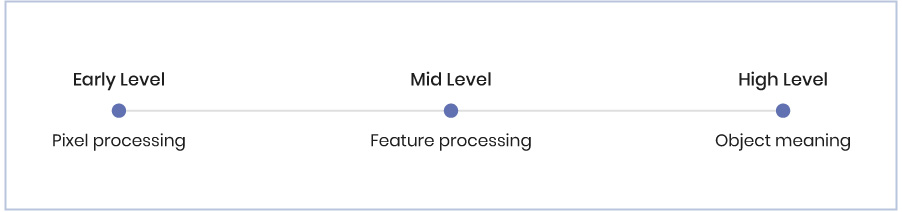According to OpenCV homepage, OpenCV is:

OpenCV (Open Source Computer Vision Library) is released under a BSD license and hence it’s free for both academic and commercial use. It has C++, Python and Java interfaces and supports Windows, Linux, Mac OS, iOS and Android. OpenCV was designed for computational efficiency and with a strong focus on real-time applications. Written in optimized C/C++, the library can take advantage of multi-core processing. Enabled with OpenCL, it can take advantage of the hardware acceleration of the underlying heterogeneous compute platform.

For installation and settings, you can refer to this article on MacOS. Basically, you’ll need Python, OpenCV library for Python, numpy and matplotlib.

## 1. Basic definitions and theories

### Image representation

Image representation is an image of dimensions. M×N is defined by the function b with:
b: DM x DN → In
Where DM = {1, 2, …, M}, DN = {1, 2, …, N} and n = 1, 2, 3,…

### Pixel

The smallest part of an image is the pixel so we can call it a picture element. It is characterized by its position (i, j) and the intensity vector b(i, j).

### Image histogram

If we represent an image in black-and-white color, each pixel in the image has a value that represents the amount of light. It only stands for intensity information.
So, if we have a digital image with a gray level in the range of [0, L-1]. A histogram is a plot that shows the “probability” p(rk) of the occurrence of gray- level rk with:
p(rk) = nk/N

• rk is the kth gray level
• nk is number of pixels with kth gray level
• N is total number of pixels
• k = 0, 1, 2, 3, 4, 5, …, L-1

So we can call it the “gray level distribution.”Example of a histogram

Histograms reveal a lot about an image. We will dive deeper into that later.

## 1. Applications

### First steps

We will verify if you are ready to work with OpenCV. First, create a .py file and import the necessary libraries like below:

```import cv2
import matplotlib
import numpy```

If you don’t have any problems running this file then you are ready to continue.
Now, to have a very first application of OpenCV, we will first start with the histogram of an image below.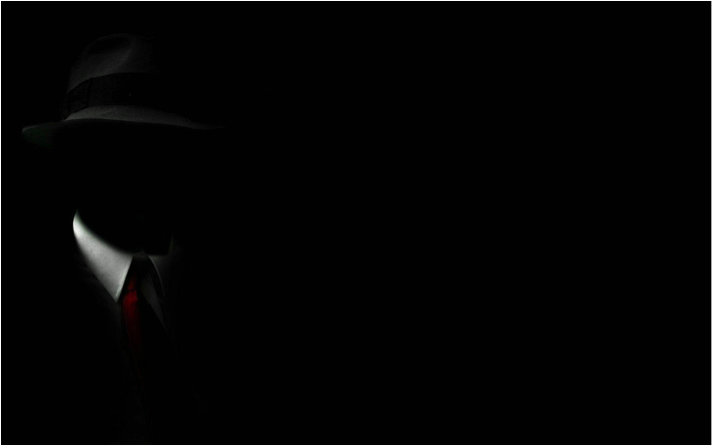This image is named “gentleman.jpg”, use the below code to extract the histogram.

```import cv2
import numpy as np
from matplotlib import pyplot as plt
plt.hist(img.ravel(),256,[0,256])
plt.show()```

The image has a lot of dark-color parts. This is reflected in the chart as you can see the number of pixels with low level of light is very high. The result: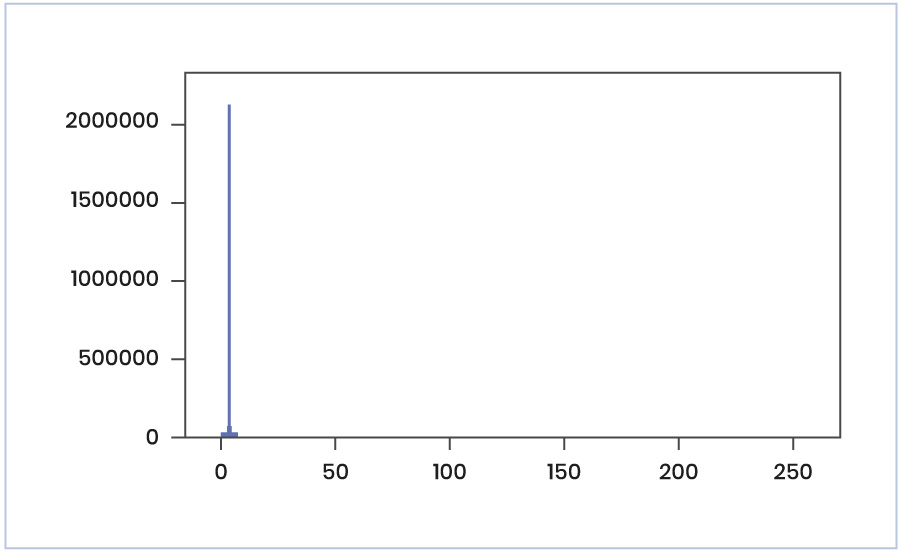### Gamma correction

Correction (Enhancement) is to make an image more suitable than the original one for a specific application.
Gamma correction method is useful when you want to change the contrast and brightness of an image.
To understand about gamma correction, first we need to understand about Power Law Transformation. With input images f(x, y), and after transformation T, we have the enhanced output image g(x, y):
g(x, y) = T[ f(x, y) ]
If we denote r, s as the gray level of f(x, y) and g(x, y) for any point (x, y), the formula can be written as: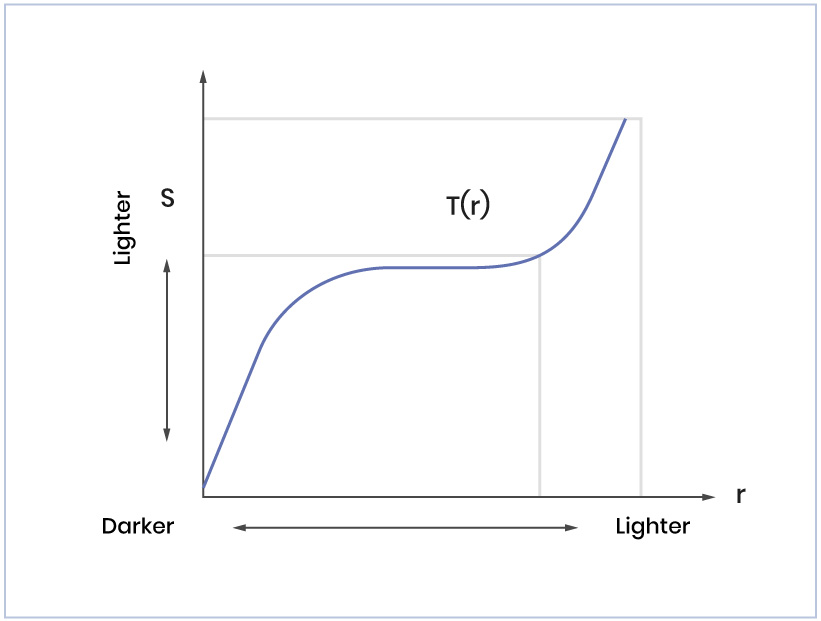s = T(r)

An example of T

In the above example, for any gray level value, in the x-axis, we have the corresponding value in the y-axis as the result of the transformation.
So, to enhance the brightness of the image, we will change the value r to reach the value s. The Power Law Transformation is defined to do the work, and its form is:
s = c*ry
The above transformation uses r power γ (gamma), so it is called Power Law Transformation. By changing the value of γ, we have different results. So the gamma correction is the process of choosing the best value for gamma to have the best output image.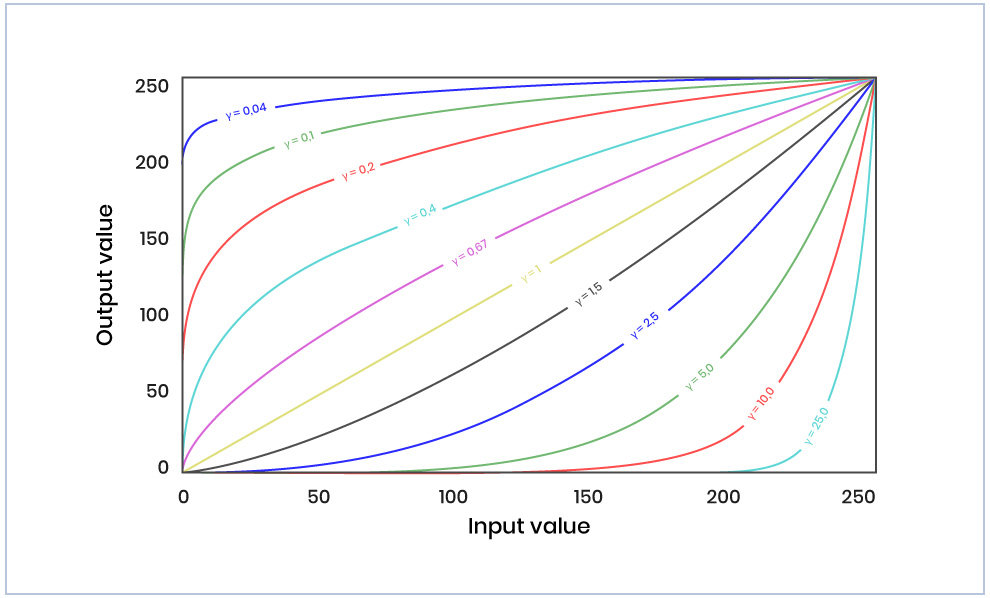Plot for different values of gamma

When γ < 1, the original dark regions will be brighter and the histogram will be shifted to the right whereas it will be the opposite with γ > 1.
For this demonstration, we will use a really dark photo like the one below: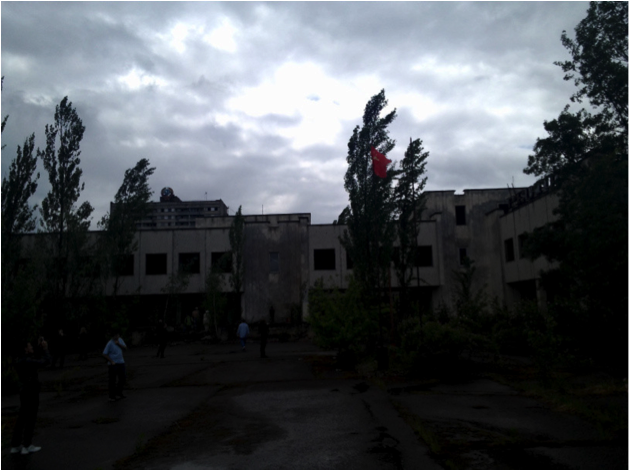We will use gamma correction method to make it have a better brightness:

```import cv2
import numpy as np
from matplotlib import pyplot as plt
plt.imshow(darkImage)
plt.show()
table = np.array([((i / 255.0) ** gamma) * 255
for i in np.arange(0, 256)]).astype("uint8")
# apply gamma correction using the lookup table
return cv2.LUT(image, table)
plt.show()```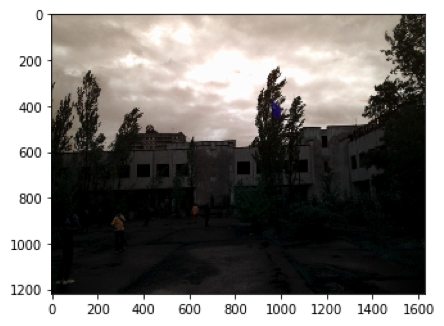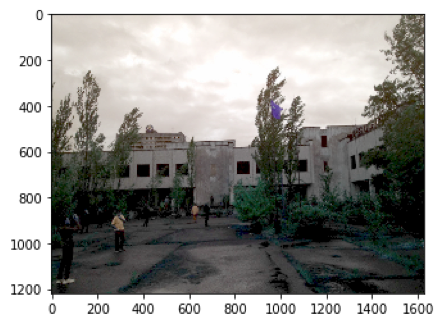Take note of the difference. It’s like magic, right? The result:

### Thresholding

The idea behind thresholding is really simple. At the most basic level, thresholding is used  to convert everything to white or black, based on a threshold value. By making use of the gray level, if the threshold is 125 (out of 255), then any value that was 125 and under would be converted to 0 (it means black), and everything above 125 would be converted to 255 (it means white). Normally, we will convert the input image to gray-scale before applying threshold.
In order to demonstrate this method, let’s look at a really dark book page like the one below:As you can see, the background really has no white in it at all. Everything is dim, but also everything is varying. On the left side, we have enough light to read the text, while the rest of the image is quite dark and requires a bit of focus to even try to read the text.
So, in order to apply the threshold, we use THRESH_BINARY threshold type. The function transforms a grayscale image to a binary image according to the formula below:For the method ADAPTIVE_THRESH_GAUSSIAN_C , the threshold value T(x, y) is a weighted sum (cross-correlation with a Gaussian window) of the blockSize x blockSize neighborhood of (x, y) minus C . The default sigma (standard deviation) is used for the specified blockSize. So we have the code:

```import cv2
import numpy as np
from matplotlib import pyplot as plt
plt.imshow(img)
plt.show()
grayscaled = cv2.cvtColor(img,cv2.COLOR_BGR2GRAY)